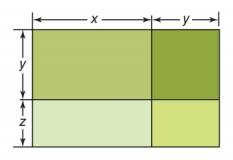Chapter A.1, Problem 28EElementary Geometry For College St...

7th Edition
Alexander + 2 others
ISBN: 9781337614085

Solutions

Chapter
SectionElementary Geometry For College St...

7th Edition
Alexander + 2 others
ISBN: 9781337614085
Textbook Problem

The area of an enclosed plot of ground that a farmer has subdivided can be found by multiplying ( x + y ) times ( y + z ) . Use FOIL to complete the multiplication. How does this product compare with the total of the areas of the four smaller plots?To determine

To find:

The area of the enclosed plot of ground using FOIL method.

Explanation

1) In FOIL method, First, Outside, Inside, and Last pairs of terms are multiplied and then added.

2) The area of the rectangle is given by LW where L is the length and W is width of the rectangle.

Calculation:

It is given that the area of the enclosed plot is (x+y) times (y+z).

Apply FOIL to multiply (x+y) by (y+z)

(x+y)(y+z)

Still sussing out bartleby?

Check out a sample textbook solution.

See a sample solution

The Solution to Your Study Problems

Bartleby provides explanations to thousands of textbook problems written by our experts, many with advanced degrees!

Get Started

Describe the set of points (x,y) such that x2+y2=9.

Finite Mathematics and Applied Calculus (MindTap Course List)

In Exercises 1-4, simplify the expression. x316xx3+2x28x

Calculus: An Applied Approach (MindTap Course List)

Reduce to lowest terms. 8.

Contemporary Mathematics for Business & Consumers

Use the Taylor polynomial of degree 2 for centered at 1 to estimate . 1.250 1.255 1.265 1.270

Study Guide for Stewart's Single Variable Calculus: Early Transcendentals, 8th

The equation of the tangent plane to for is:

Study Guide for Stewart's Multivariable Calculus, 8th